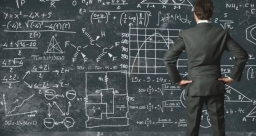# The whole number

A whole number is increased by seven, and the obtained number is multiplied by five. The result is 45. Find this number.

x =  2

### Step-by-step explanation:Did you find an error or inaccuracy? Feel free to write us. Thank you!

Tips for related online calculators
Do you have a linear equation or system of equations and looking for its solution? Or do you have a quadratic equation?